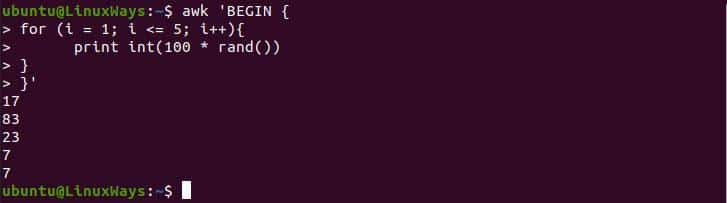# 10 Awesome Awk Command Examples in LinuxAwk is the most popular utility that is developed for the purpose of data extraction, text processing, and moreover like creating formatted reports. It is way more similar to sed but more powerful than sed as sed has limitations in text processing. AWK doesn’t have a specific meaning to its name as it is named using the first letter of its developers Alfred Aho, Peter J. Weinberger, and Brian Kernighan.

In this article, we will learn 10 awesome awk commands you must need to know. I have created and added the following set of data in student.txt as an example. The data set has 4 columns where the first field contains the first name, the second field contains the second name, the third field contains age and the last one contains the class.#### Printing Specific Field Using Variable

Awk has many prebuilt variables that have their respective purpose. Using this command we can print all the specific field data using \$x where x refers to the field numbering position.

`\$ awk '{print \$1, \$2}' student.txt`#### BEGIN Variable

BEGIN Variable is used to add header or title to resulting data as it executed the script before processing the data. It helps in indexing while formatting the data tables. In the following example, I have printed some text as indexing and then print all student names.

`\$ awk 'BEGIN {print "Students : "} {print \$1}' student.txt`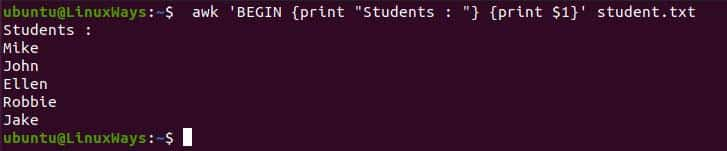#### END Variable

END is just the opposite of BEGIN as it executes the script after data processing. It can be used for the final reporting of the data set. In the following example, I have printed all the student age and then printed some ending messages.

```\$ awk '{print \$3}

END {

print "These are student age "

} ' student.txt```#### File Separator

Space and Tab space are default separators of the awk command however we can separate text based on other separators like comma, slash, etc. To achieve this we need to add the -F flag to the command and the provide separator in a single quotation mark.

`\$ awk -F':' '{print \$1}' /etc/passwd`#### Running Script From File

We can execute the awk script from the file also which provides us the tendency of creating reports efficiently. For this, you need to create the file then write the script and execute it using the awk command. For the demo, you can create a file name demo_script and copy-paste the following script.

```\$ vi demo_script

{

sum+=\$3

}

END {

print("Sum of all student age is", sum)

}```The awk command provides a -f flag for executing the script from the file.

`\$ awk -f demo_script student.txt`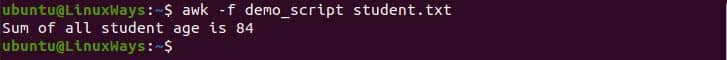#### Using Multiple Script

We can execute the multiple scripts using the semicolon. In the following example, I have printed some text then pipe the output, with awk and print out the modified result.

`\$ echo "Hello, Dr. John" | awk '{\$3="George"; print \$0}'`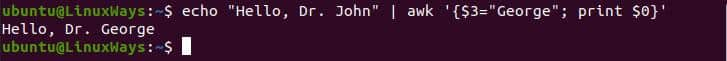#### Count Number of Lines

We can allocate the number to the report using the NR variable which is awk built-in variable that automatically prints the line number to the report.

`\$ awk '{print NR "\t" \$0}' student.txt`#### Count Number of Fields

Sometimes, while preparing the data we forgot to add data in the specific column which may lead to irregularity in the report. We can count fields using the NF variable which makes us easier to review and arrange the reports.

`\$ awk '{print NR".",\$0 "\n Count=" NF}' student.txt`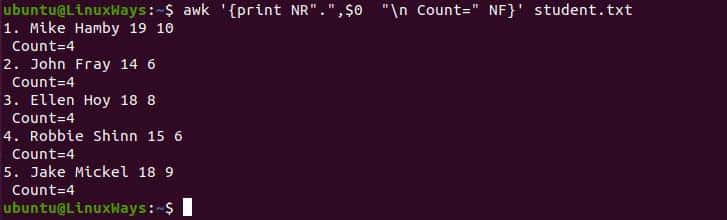#### If Condition

We can use if condition in preparing a conditional report. In the following example, we print all the student whose age is below 16

```\$ awk '

BEGIN{

print "Student whose age are under 16 are:"

}

{

if(\$3<16){

print \$1

}

}' student.txt```#### For Loop

In the following example, we use for loop to print 5 random numbers in succession. For generating random numbers we will use the rand() function which is a system inbuilt function. This function will generate a random number in decimal so we need to multiply 100 to get random numbers 1 to 100.

```\$ awk 'BEGIN {

for (i = 1; i <= 5; i++){

print int(100 * rand())

}

}'```